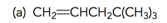# Problem: Draw a bond-line structure for each of the following compounds:

###### Problem Details

Draw a bond-line structure for each of the following compounds:What scientific concept do you need to know in order to solve this problem?

Our tutors have indicated that to solve this problem you will need to apply the Condensed Structural Formula concept. You can view video lessons to learn Condensed Structural Formula. Or if you need more Condensed Structural Formula practice, you can also practice Condensed Structural Formula practice problems.

What is the difficulty of this problem?

Our tutors rated the difficulty ofDraw a bond-line structure for each of the following compoun...as medium difficulty.

What professor is this problem relevant for?

Based on our data, we think this problem is relevant for Professor Cruz's class at USF.

What textbook is this problem found in?

Our data indicates that this problem or a close variation was asked in Organic Chemistry - Klein 1st Edition. You can also practice Organic Chemistry - Klein 1st Edition practice problems.